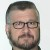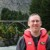# Understanding Permutations and Combinations

ByLew Yerian

In Six Sigma problem solving, it is often important to calculate the likelihood that a combination of events or an ordered combination of events will occur. Understanding some of the basic concepts of probability provides practitioners with the tools to make predictions about events or event combinations. This provides a good foundation for understanding probability distributions, confidence intervals and hypothesis testing. Permutations and combinations are two important concepts for building this foundation.

But, permutations and combinations cause a lot of confusion: “Which one is which?” and “Which one do I use?” are common questions.

### Permutations

If I purchase a salad for lunch, it may be a mix of lettuce, tomatoes, carrots and radishes. I don’t really care what order the vegetables are when they are placed in the bowl. All that I care about is that I have a salad that contains lettuce, tomatoes, carrots and radishes. The salad could consist of “carrots, tomatoes, radishes and lettuce” or “radishes, tomatoes, carrots and lettuce.” It’s still the same salad to me.

How about the PIN for my bank account? “The PIN to my account is 8-9-10.” If I want to access my bank account through the ATM, I do need to care about the order of those numbers. “10-9-8” would not access my account. Neither would “9-10-8.” It has to be exactly 8-9-10. The order is important.

Details matter for permutations – every little detail. To a permutation, red/yellow/green is different from green/yellow/red. Order is important and absolutely must be preserved.

### Combinations

Combinations are much easier to get along with – details don’t matter so much. To a combination, red/yellow/green looks the same as green/yellow/red.

Permutations are for lists (where order matters) and combinations are for groups (where order doesn’t matter). In other words: A permutation is an ordered combination.

Handpicked Content:   Spreading Success at Colorado Department of Transportation

Note: A “combination” lock should really be called a “permutation” lock because the order that you put the numbers in matters. A true “combination” lock would open using either 10-17-23 or 23-17-10. Actually, any combination of 10, 17 and 23 would open a true “combination” lock.

### Permutations: Details, Details, Details

Permutations are all possible ways of arranging the elements of a set. We’re going to be concerned about every last detail, including the order of each item. Permutations see differently ordered arrangements as different answers.
Let’s say that we have five people in a barbecue contest: Andy, Bob, Charlie, David and Eric.

How many ways can we award the first, second and third place ribbons (blue, red and yellow) among the 5 contestants?Since the order in which ribbons are awarded is important, we need to use permutations.

Here’s a breakdown:

• Blue ribbon: There are five choices: A B C D E. Let’s say A wins the blue ribbon.
• Red ribbon: There are four remaining choices: B C D E. Let’s say B wins the red ribbon.
• Yellow ribbon: There are three remaining choices: C D E. Let’s say C wins the yellow ribbon.

For this example, we picked certain people to win, but that doesn’t really matter. All that matters is that we understand that we had five choices at first, then four and then three. The total number of options was 5 × 4 × 3 = 60. We had to order three people out of five. To do this, we started with all five options then took them away one at a time (four, then three, etc.) until we ran out of ribbons.

Five-factorial (written 5!) is: 5! = 5 × 4 × 3 × 2 × 1 = 120.

But 120 is too big! It would work if we had five ribbons. But we don’t; we have three ribbons. We only want 5 × 4 × 3 (the total number of options). How do we get the factorial to “stop” at 3? We need to get rid of the 2 × 1. What do we call 2 × 1? 2-factorial! This is what is left over after we pick three winners from five contestants.

Handpicked Content:   CCPM and Lean / Six Sigma

If we divide 5! by 2!, we get: 5! / 2! = (5 × 4 × 3 × 2 × 1) / (2 × 1) = 5 × 4 × 3 = 60 (because the 2 × 1 in the numerator and denominator will cancel each other out).

A better (simpler) way to write this would be: 5! / (5 – 3)!

This is saying, “use the first three numbers of 5!”

In more general terms, if we have n items total and want to pick k in a certain order, we get:
n! / (n – k)!

And this is the permutation formula: The number of ways k items can be ordered from n items:
P(n,k) = n! / (n – k)!

### Combinations: No Order Needed

We all have a relative that is laid back. Nothing bothers them. They just go about their lives as if nothing really matters.

Combinations are the happy-go-lucky cousins of permutations. Order doesn’t matter to them. You can mix the order up, and they’re still happy. The world looks the same to them whether it’s ordered or not ordered.

Let’s say that instead of awarding blue, red and yellow ribbons for our barbecue contest, we award the top three with “participant” ribbons. How many ways can I award three participant ribbons to five people?

In this case, the order doesn’t matter. If I give a participant ribbon to Andy, Bob and Charlie, it’s the same as giving a participant ribbon to Charlie, Andy and Bob. Either way, they’re all going to be equally disappointed.

Wait a minute! Andy/Bob/Charlie = Charlie/Bob/Andy. We’ve got some repeats here. Ignore that for a moment, let’s just figure out how many ways we can rearrange three people.

Handpicked Content:   Selecting Black Belts

We have three choices for the first person, two for the second and only one for the last. So we have 3 * 2 * 1 ways to re-arrange three people.

But this looks like a permutation – it is! If you have N people and you want to know how many arrangements there are for all of them, it’s just N-factorial or N!

So, if we have three participant ribbons to give away, there are 3! equals six variations for every choice we pick. If we want to figure out how many combinations we have, we create all of the permutations and divide by all of the redundancies. In our case, we get 336 permutations (8 x 7 x 6), and we divide by the six redundancies for each permutation and get 336/6 = 56.

Therefore, the general formula for a combination is:
C(n,k) = P(n,k) / k!

This means: “Find all the ways to pick k people from n, and divide by the k! variants.” Writing this out, we get our combination formula, or the number of ways to combine k items from a set of n:
C(n,k) = n! / (n-k)! × k!

### Conclusion

It’s more important to understand why permutations and combinations work than it is to memorize the formulas. You can always look up the formulas if you forget them.

Just remember:

• If the order doesn’t matter, it is a combination.
• If the order does matter it is a permutation.
##### Lew Yerian

1.Chris Seider

nicely and simply written…

2.sarim

Another example is:
Permutations: How many ways ‘r’ kids can be picked out of ‘n’ kids and arranged in a line. There are ‘r’ positions in a line.
Any of the n kids can be put in position 1. So there are n choices for position 1 which is n-+1 i.e. n-1+1.
Any of the remaining (n-1) kids can be put in position 2. So there are n-1 choices for position 2 which is n-+1 i.e. n-2+1.
Similarly there are n-2 choices for position 3 which is n-+1 i.e. n-3+1
And there will be n-+1 choices for rth position. n-r+1. So the total number of ways ‘r’ kids can be picked out of ‘n’ kids and arranged in a line is n*(n-1)*(n-2)*….(n-r+1) = (n!)/ (n-r)!
This is written as P(n,r).
Combination: How many ways ‘r’ kids can be picked out of ‘n’ and just put in a group i.e. no arranging them in a line. So once ‘r’ kids are picked out, it is just considered a single choice.
In the first case, after picking ‘r’ kids we arranged them in line. Each of these arrangements generated a new arrangement. All these arrangements are counted as one one for the second case. Let’s see how many arrangement of ‘r’ kids can be done when we put them in a line.
There are r choices for the first position.
r-1 choices for the 2nd position.
r-2 choices for the 3rd position.
..
1 choice for the rth position.
So after picking ‘r’ kids, we can arrange them in a line in r! ways. All these ways should be counted as one. So
the formula for combination (just picking r kids but not arranging them in a line)
(Number of ways for arranging r kids in a line out of n kids total) ÷ (number of ways of arranging r kids in a line)
[(n!)/ (n-r)!] ÷ r! = (n!)/ [(n-r)!*(r!)]

3.Ainerukundo Dickson

it was so much helpful to me
thanks